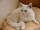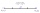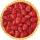# Trees avg

The worker planted 96 trees on Monday, 120 on Tuesday and 61 trees on Wednesday. How many trees did he plant on Thursday if he averaged 105 trees per day?

Result

x =  143

#### Solution:

(96+120+61+x)/4 = 105

x = 143

x = 143

Calculated by our simple equation calculator.Our examples were largely sent or created by pupils and students themselves. Therefore, we would be pleased if you could send us any errors you found, spelling mistakes, or rephasing the example. Thank you!

Leave us a comment of this math problem and its solution (i.e. if it is still somewhat unclear...):Be the first to comment!#### Following knowledge from mathematics are needed to solve this word math problem:

Looking for help with calculating arithmetic mean? Looking for a statistical calculator? Do you have a linear equation or system of equations and looking for its solution? Or do you have quadratic equation?

## Next similar math problems:

1. CatOne-fifth of the monthly pocket money contributes Maros for food for his cat, half of the rest postpone for a new smartphone. The remaining € 8 spend. How much pocket money gets Maros a month?
2. CandyPeter had a sachet of candy. He wanted to share with his friends. If he gave them 30 candies, he would have 62 candies. If he gave them 40 candies, he would miss 8 candies. How many friends did Peter have?
3. CakesGrandmother baked cakes. Half of its was poppy, quarter with plum jam and 16 cheesecakes. How many cakes she baked in total?
4. Boys and girlsThere are 48 children in the sports club, boys are 10 more than girls. How many girls go to the club?
5. Spain vs USASpain lost to the US by 4 goals. In the match total fell 10 goals. How many goals gave the Spain and how the United States?
6. Two trainsThere were 159 freight wagons on the railway station creating 2 trains. One had 15 more wagons than the other. How many wagons did each train have?
7. Simple equationSolve for x: 3(x + 2) = x - 18
8. EquationSolve the equation: 1/2-2/8 = 1/10; Write the result as a decimal number.
9. AverageThe arithmetic mean of the two numbers is 71.7. One number is 5. Calculate the second number.
10. Unknown numberIdentify unknown number which 1/5 is 40 greater than one tenth of that number.
11. Arithmetic averageThe arithmetic mean of the five numbers is exactly 8. The sum of these four numbers is 30. What is the fifth number?
12. Find midpointFM=5y+13, MG=5-3y, FG=? M is the midpoint of FG. Use the given information to find the missing measure or value.
13. Strange xFor what x is true ??
14. One-thirdA one-third of unknown number is equal to five times as great as the difference of the same unknown number and number 28. Determine the unknown number.
15. UN 1If we add to an unknown number his quarter, we get 210. Identify unknown number.
16. GardenThe garden around the new majer is divided as follows: 35% vegetable, 30% fruit orchards, 10% flowers and the remaining 120 m2 are lawns. What is the total area of the garden?
17. FractionsThree-quarters of an unknown number are 4/5. What is 5/6 of this unknown number?